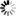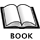Normal view

## Discovering statistics using IBM SPSS Statistics / Andy Field

Publisher: Los Angeles (USA) : SAGE, 2018Edition: 5th edDescription: 1070 p. : il. col. ; 27 cmISBN: 9781526419514; 9781526419521Summary: Chapter 1: Why is my evil lecturer forcing me to learn statistics? Chapter 2: The SPINE of statistics Chapter 3: The phoenix of statistics Chapter 4: The IBM SPSS Statistics environment Chapter 5: Exploring data with graphs Chapter 6: The beast of bias Chapter 7: Non-parametric models Chapter 8: Correlation Chapter 9: The Linear Model (Regression) Chapter 10: Comparing two means Chapter 11: Moderation, mediation and multicategory predictors Chapter 12: GLM 1: Comparing several independent means Chapter 13: GLM 2: Comparing means adjusted for other predictors (analysis of covariance) Chapter 14: GLM 3: Factorial designs Chapter 15: GLM 4: Repeated-measures designs Chapter 16: GLM 5: Mixed designs Chapter 17: Multivariate analysis of variance (MANOVA) Chapter 18: Exploratory factor analysis Chapter 19: Categorical outcomes: chi-square and loglinear analysis Chapter 20: Categorical outcomes: logistic regression Chapter 21: Multilevel linear modelsAverage rating: 0.0 (0 votes)
Item type Current location Collection Call number Status Date due Barcode Course reservesLibro Biblioteca Universidad Europea del Atlántico
Fondo General
No ficción 519.22 FIE dis Available 4368

Chapter 1: Why is my evil lecturer forcing me to learn statistics?
Chapter 2: The SPINE of statistics
Chapter 3: The phoenix of statistics
Chapter 4: The IBM SPSS Statistics environment
Chapter 5: Exploring data with graphs
Chapter 6: The beast of bias
Chapter 7: Non-parametric models
Chapter 8: Correlation
Chapter 9: The Linear Model (Regression)
Chapter 10: Comparing two means
Chapter 11: Moderation, mediation and multicategory predictors
Chapter 12: GLM 1: Comparing several independent means
Chapter 13: GLM 2: Comparing means adjusted for other predictors (analysis of covariance)
Chapter 14: GLM 3: Factorial designs
Chapter 15: GLM 4: Repeated-measures designs
Chapter 16: GLM 5: Mixed designs
Chapter 17: Multivariate analysis of variance (MANOVA)
Chapter 18: Exploratory factor analysis
Chapter 19: Categorical outcomes: chi-square and loglinear analysis
Chapter 20: Categorical outcomes: logistic regression
Chapter 21: Multilevel linear models

Share

Servicio de Biblioteca de la Universidad Europea del Atlantico | biblioteca@uneatlantico.es | Tlf: 942 244 244 Ext. 5020